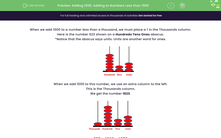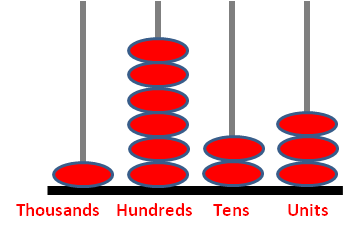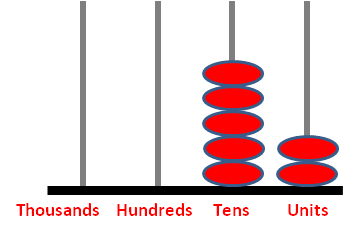# Add 1,000 to Numbers that are Less than 1,000

In this worksheet, students will add 1,000 to a number less than 1,000.Key stage:  KS 2

Curriculum topic:   Number: Number and Place Value

Curriculum subtopic:   Find 1000 More or Less than a Given Number

Popular topics:   Subtraction worksheets, Addition worksheets

Difficulty level:#### Worksheet Overview

When we add 1,000 to a number less than a thousand, we must place a 1 in the thousands column.

Here is the number 623 shown on an abacus.

Notice that the abacus says units. Units are another word for ones.When we add 1,000 to this number, we add an extra column to the left.

This is the thousands column.

We get the number 1,623.623 + 1,000 = 1,623

Don't forget zeros!

Here is 52 shown on an abacus.When we add 1,000, we get:Notice that there are no hundreds.

This number is 1,052.

52 + 1,000 = 1,052

Putting a zero in the hundreds column is really important to act as a place holder. Without the zero, the number we would have got would have been 152, which is very different to 1,052, isn't it!### What is EdPlace?

We're your National Curriculum aligned online education content provider helping each child succeed in English, maths and science from year 1 to GCSE. With an EdPlace account you’ll be able to track and measure progress, helping each child achieve their best. We build confidence and attainment by personalising each child’s learning at a level that suits them.

Get started